# Tag: Connes

The monstrous moonshine picture is the finite piece of Conway’s Big Picture needed to understand the 171 moonshine groups associated to conjugacy classes of the monster.

Last time I claimed that there were exactly 7 types of local behaviour, but I missed one. The forgotten type is centered at the number lattice $84$.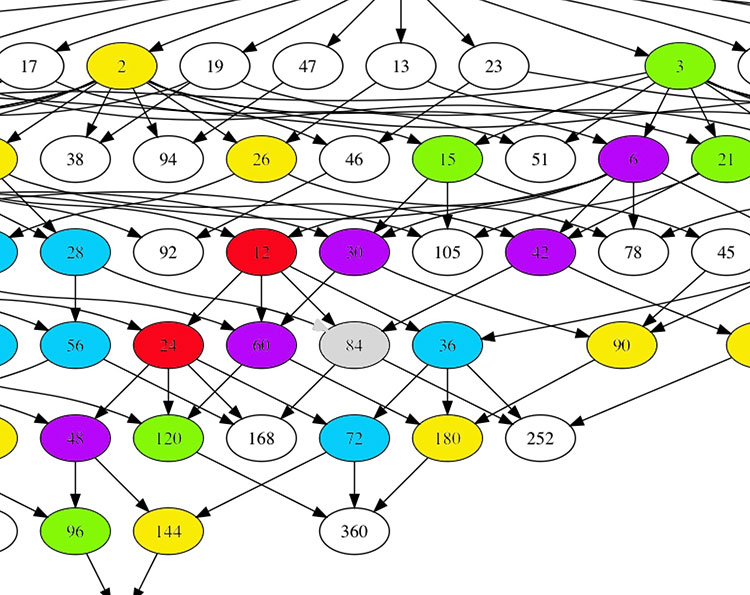Locally around it the moonshine picture looks like this
$\xymatrix{42 \ar@{-}[dr] & 28 \frac{1}{3} \ar@[red]@{-}[d] & 41 \frac{1}{2} \ar@{-}[ld] \\ 28 \ar@[red]@{-}[r] & \color{grey}{84} \ar@[red]@{-}[r] \ar@[red]@{-}[d] \ar@{-}[rd] & 28 \frac{2}{3} \\ & 252 & 168}$

and it involves all square roots of unity ($42$, $42 \frac{1}{2}$ and $168$) and $3$-rd roots of unity ($28$, $28 \frac{1}{3}$, $28 \frac{2}{3}$ and $252$) centered at $84$.

No, I’m not hallucinating, there are indeed $3$ square roots of unity and $4$ third roots of unity as they come in two families, depending on which of the two canonical forms to express a lattice is chosen.

In the ‘normal’ expression $M \frac{g}{h}$ the two square roots are $42$ and $42 \frac{1}{2}$ and the three third roots are $28, 28 \frac{1}{3}$ and $28 \frac{2}{3}$. But in the ‘other’ expression
$M \frac{g}{h} = (\frac{g’}{h},\frac{1}{h^2M})$
(with $g.g’ \equiv 1~mod~h$) the families of $2$-nd and $3$-rd roots of unity are
$\{ 42 \frac{1}{2} = (\frac{1}{2},\frac{1}{168}), 168 = (0,\frac{1}{168}) \}$
and
$\{ 28 \frac{1}{3} = (\frac{1}{3},\frac{1}{252}), 28 \frac{2}{3} = (\frac{2}{3},\frac{1}{252}), 252 = (0 , \frac{1}{252}) \}$
As in the tetrahedral snake post, it is best to view the four $3$-rd roots of unity centered at $84$ as the vertices of a tetrahedron with center of gravity at $84$. Power maps in the first family correspond to rotations along the axis through $252$ and power maps in the second family are rotations along the axis through $28$.

In the ‘normal’ expression of lattices there’s then a total of 8 different local types, but two of them consist of just one number lattice: in $8$ the local picture contains all square, $4$-th and $8$-th roots of unity centered at $8$, and in $84$ the square and $3$-rd roots.

Perhaps surprisingly, if we redo everything in the ‘other’ expression (and use the other families of roots of unity), then the moonshine picture has only 7 types of local behaviour. The forgotten type $84$ appears to split into two occurrences of other types (one with only square roots of unity, and one with only $3$-rd roots).

I wonder what all this has to do with the action of the Bost-Connes algebra on the big picture or with Plazas’ approach to moonshine via non-commutative geometry.

All lattices in the moonshine picture are number-like, that is of the form $M \frac{g}{h}$ with $M$ a positive integer and $0 \leq g < h$ with $(g,h)=1$. To understand the action of the Bost-Connes algebra on the Big Picture it is sometimes better to view the lattice $M \frac{g}{h}$ as a primitive $h$-th root of unity, centered at $hM$.

The distance from $M$ to any of the lattices $M \frac{g}{h}$ is equal to $2 log(h)$, and the distances from $M$ and $M \frac{g}{h}$ to $hM$ are all equal to $log(h)$.

For a prime value $h$, these $h$ lattices are among the $h+1$ lattices branching off at $hM$ in the $h$-adic tree (the remaining one being $h^2M$).

For general $h$ the situation is more complex. Here’s the picture for $h=6$ with edges in the $2$-adic tree painted blue, those in the $3$-adic tree red.

$\xymatrix{& & M \frac{1}{2} \ar@[blue]@{-}[d] & \\ & M \ar@[blue]@{-}[r] \ar@[red]@{-}[d] & 2M \ar@[red]@{-}[d] & M \frac{1}{6} \ar@[red]@{-}[d] \\ M \frac{1}{3} \ar@[red]@{-}[r] & 3M \ar@[blue]@{-}[r] \ar@[red]@{-}[d] & \boxed{6 M} \ar@[blue]@{-}[r] & 3M \frac{1}{2} \ar@[red]@{-}[d] \\ & M \frac{2}{3} & & M \frac{5}{6}}$

To describe the moonshine group $(n|h)+e,f,\dots$ (an example was worked out in the tetrahedral snake post), we need to study the action of base-change with the matrix
$x = \begin{bmatrix} 1 & \frac{1}{h} \\ 0 & 1 \end{bmatrix}$
which sends a lattice of the form $M \frac{g}{h}$ with $0 \leq g < h$ to $M \frac{g+M}{h}$, so is a rotation over $\frac{2 \pi M}{h}$ around $h M$. But, we also have to describe the base-change action with the matrix $y = \begin{bmatrix} 1 & 0 \\ n & 1 \end{bmatrix}$ and for this we better use the second description of the lattice as $M \frac{g}{h}=(\frac{g'}{h},\frac{1}{h^2M})$ with $g'$ the multiplicative inverse of $g$ modulo $h$. Under the action by $y$, the second factor $\frac{1}{h^2M}$ will be fixed, so this time we have to look at all lattices of the form $(\frac{g}{h},\frac{1}{h^2M})$ with $0 \leq g < h$, which again can be considered as another set of $h$-th roots of unity, centered at $hM$. Here's this second interpretation for $h=6$: $\xymatrix{M \frac{5}{6} \ar@[red]@{-}[d] & & 4M \frac{1}{3} \ar@[red]@{-}[d] & \\ 3M \frac{1}{2} \ar@[blue]@{-}[r] \ar@[red]@{-}[d] & \boxed{6M} \ar@[blue]@{-}[r] \ar@[red]@{-}[d] & 12 M \ar@[red]@{-}[r] \ar@[red]@{-}[d] & 4 M \frac{2}{3} \\ M \frac{1}{6} & 18 M \ar@[blue]@{-}[r] \ar@[blue]@{-}[d] & 36 M & \\ & 9M \frac{1}{2} & & }$ Under $x$ the first set of $h$-th roots of unity centered at $hM$ is permuted, whereas $y$ permutes the second set of $h$-th roots of unity.
These interpretations can be used to spot errors in computing the finite groups $\Gamma_0(n|h)/\Gamma_0(n.h)$.

Here’s part of the calculation of the action of $y$ on the $(360|1)$-snake (which consists of $60$-lattices).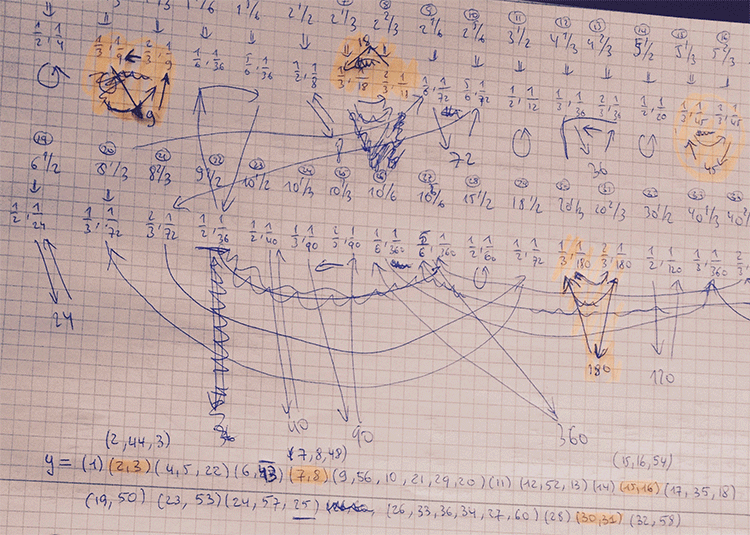First I got a group of order roughly $600.000$. After correcting some erroneous cycles, the order went down to 6912.

Finally I spotted that I mis-numbered two lattices in the description of $x$ and $y$, and the order went down to $48$ as it should, because I knew it had to be equal to $C_2 \times C_2 \times A_4$.

A well-known link between Conway’s Big Picture and non-commutative geometry is given by the Bost-Connes system.

This quantum statistical mechanical system encodes the arithmetic properties of cyclotomic extensions of $\mathbb{Q}$.

The corresponding Bost-Connes algebra encodes the action by the power-maps on the roots of unity.

It has generators $e_n$ and $e_n^*$ for every natural number $n$ and additional generators $e(\frac{g}{h})$ for every element in the additive group $\mathbb{Q}/\mathbb{Z}$ (which is of course isomorphic to the multiplicative group of roots of unity).

The defining equations are
$\begin{cases} e_n.e(\frac{g}{h}).e_n^* = \rho_n(e(\frac{g}{h})) \\ e_n^*.e(\frac{g}{h}) = \Psi^n(e(\frac{g}{h}).e_n^* \\ e(\frac{g}{h}).e_n = e_n.\Psi^n(e(\frac{g}{h})) \\ e_n.e_m=e_{nm} \\ e_n^*.e_m^* = e_{nm}^* \\ e_n.e_m^* = e_m^*.e_n~\quad~\text{if (m,n)=1} \end{cases}$

Here $\Psi^n$ are the power-maps, that is $\Psi^n(e(\frac{g}{h})) = e(\frac{ng}{h}~mod~1)$, and the maps $\rho_n$ are given by
$\rho_n(e(\frac{g}{h})) = \sum e(\frac{i}{j})$
where the sum is taken over all $\frac{i}{j} \in \mathbb{Q}/\mathbb{Z}$ such that $n.\frac{i}{j}=\frac{g}{h}$.

Conway’s Big Picture has as its vertices the (equivalence classes of) lattices $M,\frac{g}{h}$ with $M \in \mathbb{Q}_+$ and $\frac{g}{h} \in \mathbb{Q}/\mathbb{Z}$.

The Bost-Connes algebra acts on the vector-space with basis the vertices of the Big Picture. The action is given by:
$\begin{cases} e_n \ast \frac{c}{d},\frac{g}{h} = \frac{nc}{d},\rho^m(\frac{g}{h})~\quad~\text{with m=(n,d)} \\ e_n^* \ast \frac{c}{d},\frac{g}{h} = (n,c) \times \frac{c}{nd},\Psi^{\frac{n}{m}}(\frac{g}{h})~\quad~\text{with m=(n,c)} \\ e(\frac{a}{b}) \ast \frac{c}{d},\frac{g}{h} = \frac{c}{d},\Psi^c(\frac{a}{b}) \frac{g}{h} \end{cases}$

This connection makes one wonder whether non-commutative geometry can shed a new light on monstrous moonshine?

This question is taken up by Jorge Plazas in his paper Non-commutative geometry of groups like $\Gamma_0(N)$Plazas shows that the bigger Connes-Marcolli $GL_2$-system also acts on the Big Picture. An intriguing quote:

“Our interest in the $GL_2$-system comes from the fact that its thermodynamic properties encode the arithmetic theory of modular functions to an extend which makes it possible for us to capture aspects of moonshine theory.”

Looks like the right kind of paper to take along when I disappear next week for some time in the French mountains…

Absolute geometry is the attempt to develop algebraic geometry over the elusive field with one element $\mathbb{F}_1$. The idea being that the set of all prime numbers is just too large for $\mathbf{Spec}(\mathbb{Z})$ to be a terminal object (as it is in the category of schemes).

So, one wants to view $\mathbf{Spec}(\mathbb{Z})$ as a geometric object over something ‘deeper’, the “absolute point” $\mathbf{Spec}(\mathbb{F}_1)$.

Starting with the paper by Bertrand Toen and Michel Vaquie, Under $\mathbf{Spec}(\mathbb{Z})$, topos theory entered this topic.

First there was the proposal by Jim Borger to view $\lambda$-rings as $\mathbb{F}_1$-algebras. More recently, Alain Connes and Katia Consani introduced the arithmetic site.

Now, there are lectures series on these two approaches, one by Yuri I. Manin, the other by Alain Connes.

.

Yuri I. Manin in Ghent

On Tuesday, February 3rd, Yuri I. Manin will give the inaugural lectures of the new $\mathbb{F}_1$-seminars at Ghent University, organised by Koen Thas.

Coffee will be served from 13.00 till 14.00 at the Department of Mathematics, Ghent University, Krijgslaan 281, Building S22 and from 14.00 till 16.30 there will be lectures in the Emmy Noether lecture room, Building S25:

14:00 – 14:25: Introduction (by K. Thas)
14:30 – 15:20: Lecture 1 (by Yu. I. Manin)
15:30 – 16:20: Lecture 2 (by Yu. I. Manin)

Recent work of Manin related to $\mathbb{F}_1$ includes:

Alain Connes on the Arithmetic Site

Until the beginning of march, Alain Connes will lecture every thursday afternoon from 14.00 till 17.30, in Salle 5 – Marcelin Berthelot at he College de France on The Arithmetic Site (hat tip Isar Stubbe).

Here’s a two minute excerpt, from a longer interview with Connes, on the arithmetic site, together with an attempt to provide subtitles:

——————————————————

(50.36)

And,in this example, we saw the wonderful notion of a topos, developed by Grothendieck.

It was sufficient for me to open SGA4, a book written at the beginning of the 60ties or the late fifties.

It was sufficient for me to open SGA4 to see that all the things that I needed were there, say, how to construct a cohomology on this site, how to develop things, how to see that the category of sheaves of Abelian groups is an Abelian category, having sufficient injective objects, and so on … all those things were there.

This is really remarkable, because what does it mean?

It means that the average mathematician says: “topos = a generalised topological space and I will never need to use such things. Well, there is the etale cohomology and I can use it to make sense of simply connected spaces and, bon, there’s the chrystaline cohomology, which is already a bit more complicated, but I will never need it, so I can safely ignore it.”

And (s)he puts the notion of a topos in a certain category of things which are generalisations of things, developed only to be generalisations…

But in fact, reality is completely different!

In our work with Katia Consani we saw not only that there is this epicyclic topos, but in fact, this epicyclic topos lies over a site, which we call the arithmetic site, which itself is of a delirious simplicity.

It relies only on the natural numbers, viewed multiplicatively.

That is, one takes a small category consisting of just one object, having this monoid as its endomorphisms, and one considers the corresponding topos.

This appears well … infantile, but nevertheless, this object conceils many wonderful things.

And we would have never discovered those things, if we hadn’t had the general notion of what a topos is, of what a point of a topos is, in terms of flat functors, etc. etc.

(52.27)

——————————————————-

I will try to report here on Manin’s lectures in Ghent. If someone is able to attend Connes’ lectures in Paris, I’d love to receive updates!

Previously, we have recalled comparisons between approaches to define a geometry over the absolute point and art-historical movements, first those due to Yuri I. Manin, subsequently some extra ones due to Javier Lopez Pena and Oliver Lorscheid.

In these comparisons, the art trend appears to have been chosen more to illustrate a key feature of the approach or an appreciation of its importance, rather than giving a visual illustration of the varieties over $\mathbb{F}_1$ the approach proposes.

Some time ago, we’ve had a couple of posts trying to depict noncommutative varieties, first the illustrations used by Shahn Majid and Matilde Marcolli, and next my own mental picture of it.

In this post, we’ll try to do something similar for affine varieties over the absolute point. To simplify things drastically, I’ll divide the islands in the Lopez Pena-Lorscheid map of $\mathbb{F}_1$ land in two subsets : the former approaches (all but the $\Lambda$-schemes) and the current approach (the $\Lambda$-scheme approach due to James Borger).

The former approaches : Francis Bacon “The Pope” (1953)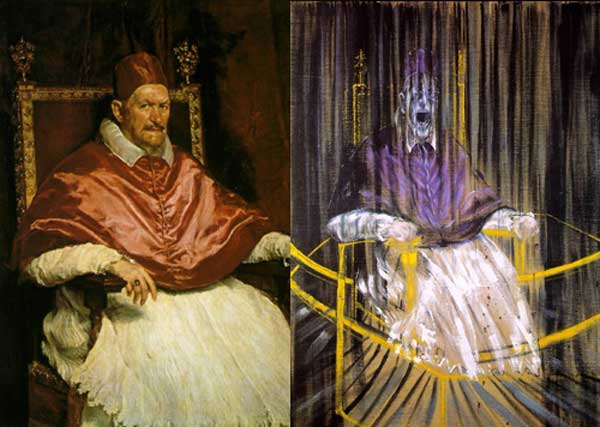The general consensus here was that in going from $\mathbb{Z}$ to $\mathbb{F}_1$ one looses the additive structure and retains only the multiplicative one. Hence, ‘commutative algebras’ over $\mathbb{F}_1$ are (commutative) monoids, and mimicking Grothendieck’s functor of points approach to algebraic geometry, a scheme over $\mathbb{F}_1$ would then correspond to a functor

$h_Z~:~\mathbf{monoids} \longrightarrow \mathbf{sets}$

Such functors are described largely by combinatorial data (see for example the recent blueprint-paper by Oliver Lorscheid), and, if the story would stop here, any Rothko painting could be used as illustration.

Most of the former approaches add something though (buzzwords include ‘Arakelov’, ‘completion at $\infty$’, ‘real place’ etc.) in order to connect the virtual geometric object over $\mathbb{F}_1$ with existing real, complex or integral schemes. For example, one can make the virtual object visible via an evaluation map $h_Z \rightarrow h_X$ which is a natural transformation, where $X$ is a complex variety with its usual functor of points $h_X$ and to connect both we associate to a monoid $M$ its complex monoid-algebra $\mathbb{C} M$. An integral scheme $Y$ can then be said to be ‘defined over $\mathbb{F}_1$’, if $h_Z$ becomes a subfunctor of its usual functor of points $h_Y$ (again, assigning to a monoid its integral monoid algebra $\mathbb{Z} M$) and $Y$ is the ‘best’ integral scheme approximation of the complex evaluation map.

To illustrate this, consider the painting Study after Velázquez’s Portrait of Pope Innocent X by Francis Bacon (right-hand painting above) which is a distorded version of the left-hand painting Portrait of Innocent X by Diego Velázquez.

Here, Velázquez’ painting plays the role of the complex variety which makes the combinatorial gadget $h_Z$ visible, and, Bacon’s painting depicts the integral scheme, build up from this combinatorial data, which approximates the evaluation map best.

All of the former approaches more or less give the same very small list of integral schemes defined over $\mathbb{F}_1$, none of them motivically interesting.

The current approach : Jackson Pollock “No. 8” (1949)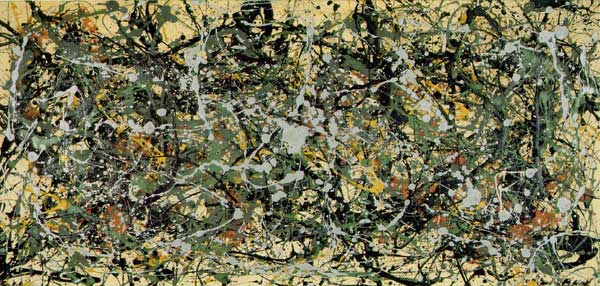An entirely different approach was proposed by James Borger in $\Lambda$-rings and the field with one element. He proposes another definition for commutative $\mathbb{F}_1$-algebras, namely $\lambda$-rings (in the sense of Grothendieck’s Riemann-Roch) and he argues that the $\lambda$-ring structure (which amounts in the sensible cases to a family of endomorphisms of the integral ring lifting the Frobenius morphisms) can be viewed as descent data from $\mathbb{Z}$ to $\mathbb{F}_1$.

The list of integral schemes of finite type with a $\lambda$-structure coincides roughly with the list of integral schemes defined over $\mathbb{F}_1$ in the other approaches, but Borger’s theory really shines in that it proposes long sought for mystery-objects such as $\mathbf{spec}(\mathbb{Z}) \times_{\mathbf{spec}(\mathbb{F}_1)} \mathbf{spec}(\mathbb{Z})$. If one accepts Borger’s premise, then this object should be the geometric object corresponding to the Witt-ring $W(\mathbb{Z})$. Recall that the role of Witt-rings in $\mathbb{F}_1$-geometry was anticipated by Manin in Cyclotomy and analytic geometry over $\mathbb{F}_1$.

But, Witt-rings and their associated Witt-spaces are huge objects, so one needs to extend arithmetic geometry drastically to include such ‘integral schemes of infinite type’. Borger has made a couple of steps in this direction in The basic geometry of Witt vectors, II: Spaces.

To depict these new infinite dimensional geometric objects I’ve chosen for Jackson Pollock‘s painting No. 8. It is no coincidence that Pollock-paintings also appeared in the depiction of noncommutative spaces. In fact, Matilde Marcolli has made the connection between $\lambda$-rings and noncommutative geometry in Cyclotomy and endomotives by showing that the Bost-Connes endomotives are universal for $\lambda$-rings.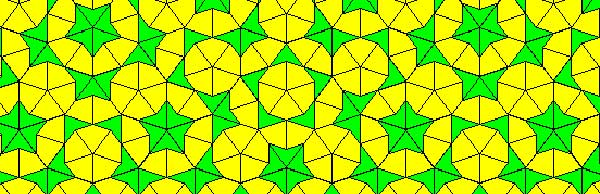Penrose tilings are aperiodic tilings of the plane, made from 2 sort of tiles : kites and darts. It is well known (see for example the standard textbook tilings and patterns section 10.5) that one can describe a Penrose tiling around a given point in the plane as an infinite sequence of 0’s and 1’s, subject to the condition that no two consecutive 1’s appear in the sequence. Conversely, any such sequence is the sequence of a Penrose tiling together with a point. Moreover, if two such sequences are eventually the same (that is, they only differ in the first so many terms) then these sequences belong to two points in the same tiling,

Another remarkable feature of Penrose tilings is their local isomorphism : fix a finite region around a point in one tiling, then in any other Penrose tiling one can find a point having an isomorphic region around it. For this reason, the space of all Penrose tilings has horrible topological properties (all points lie in each others closure) and is therefore a prime test-example for the techniques of noncommutative geometry.

In his old testament, Noncommutative Geometry, Alain Connes associates to this space a $C^*$-algebra $Fib$ (because it is constructed from the Fibonacci series $F_0,F_1,F_2,…$) which is the direct limit of sums of two full matrix-algebras $S_n$, with connecting morphisms

$S_n = M_{F_n}(\mathbb{C}) \oplus M_{F_{n-1}}(\mathbb{C}) \rightarrow S_{n+1} = M_{F_{n+1}}(\mathbb{C}) \oplus M_{F_n}(\mathbb{C}) \qquad (a,b) \mapsto ( \begin{matrix} a & 0 \\ 0 & b \end{matrix}, a)$

As such $Fib$ is an AF-algebra (for approximately finite) and hence formally smooth. That is, $Fib$ would be the coordinate ring of a smooth variety in the noncommutative sense, if only $Fib$ were finitely generated. However, $Fib$ is far from finitely generated and has other undesirable properties (at least for a noncommutative algebraic geometer) such as being simple and hence in particular $Fib$ has no finite dimensional representations…A couple of weeks ago, Paul Smith discovered a surprising connection between the noncommutative space of Penrose tilings and an affine algebra in the paper The space of Penrose tilings and the non-commutative curve with homogeneous coordinate ring $\mathbb{C} \langle x,y \rangle/(y^2)$.

Giving $x$ and $y$ degree 1, the algebra $P = \mathbb{C} \langle x,y \rangle/(y^2)$ is obviously graded and noncommutative projective algebraic geometers like to associate to such algebras their ‘proj’ which is the quotient category of the category of all graded modules in which two objects become isomorphisc iff their ‘tails’ (that is forgetting the first few homogeneous components) are isomorphic.

The first type of objects NAGers try to describe are the point modules, which correspond to graded modules in which every homogeneous component is 1-dimensional, that is, they are of the form

$\mathbb{C} e_0 \oplus \mathbb{C} e_1 \oplus \mathbb{C} e_2 \oplus \cdots \oplus \mathbb{C} e_n \oplus \mathbb{C} e_{n+1} \oplus \cdots$

with $e_i$ an element of degree $i$. The reason for this is that point-modules correspond to the points of the (usual, commutative) projective variety when the affine graded algebra is commutative.

Now, assume that a Penrose tiling has been given by a sequence of 0’s and 1’s, say $(z_0,z_1,z_2,\cdots)$, then it is easy to associate to it a graded vectorspace with action given by

$x.e_i = e_{i+1}$ and $y.e_i = z_i e_{i+1}$

Because the sequence has no two consecutive ones, it is clear that this defines a graded module for the algebra $P$ and determines a point module in $\pmb{proj}(P)$. By the equivalence relation on Penrose sequences and the tails-equivalence on graded modules it follows that two sequences define the same Penrose tiling if and only if they determine the same point module in $\pmb{proj}(P)$. Phrased differently, the noncommutative space of Penrose tilings embeds in $\pmb{proj}(P)$ as a subset of the point-modules for $P$.

The only such point-module invariant under the shift-functor is the one corresponding to the 0-sequence, that is, corresponds to the cartwheel tiling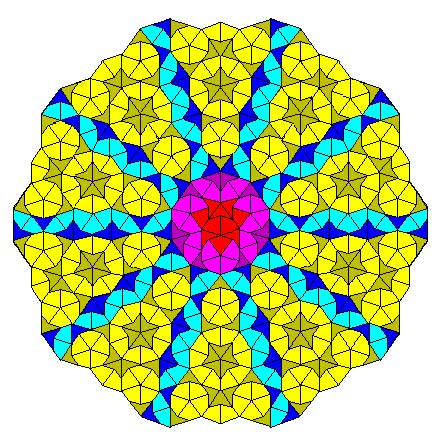Another nice consequence is that we can now explain the local isomorphism property of Penrose tilings geometrically as a consequence of the fact that the $Ext^1$ between any two such point-modules is non-zero, that is, these noncommutative points lie ‘infinitely close’ to each other.

This is the easy part of Paul’s paper.

The truly, truly amazing part is that he is able to recover Connes’ AF-algebra $Fib$ from $\pmb{proj}(P)$ as the algebra of global sections! More precisely, he proves that there is an equivalence of categories between $\pmb{proj}(P)$ and the category of all $Fib$-modules $\pmb{mod}(Fib)$!

In other words, the noncommutative projective scheme $\pmb{proj}(P)$ is actually isomorphic to an affine scheme and as its coordinate ring is formally smooth $\pmb{proj}(P)$ is a noncommutative smooth variety. It would be interesting to construct more such examples of interesting AF-algebras appearing as local rings of sections of proj-es of affine graded algebras.Last time we did recall Manin’s comparisons between some approaches to geometry over the absolute point $\pmb{spec}(\mathbb{F}_1)$ and trends in the history of art.

In the comments to that post, Javier Lopez-Pena wrote that he and Oliver Lorscheid briefly contemplated the idea of extending Manin’s artsy-dictionary to all approaches they did draw on their Map of $\mathbb{F}_1$-land.

So this time, we will include here Javier’s and Oliver’s insights on the colored pieces below in their map : CC=Connes-Consani, Generalized torified schemes=Lopez Pena-Lorscheid, Generalized schemes with 0=Durov and, this time, $\Lambda$=Manin-Marcolli.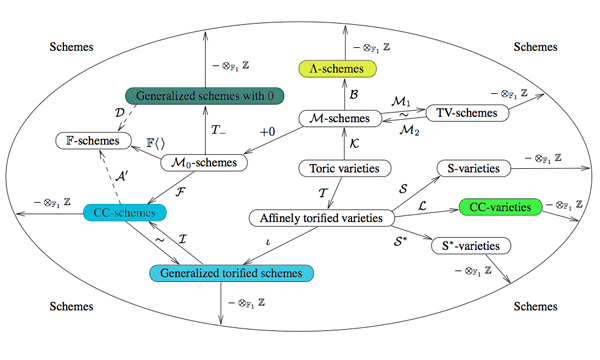Durov : romanticismIn his 568 page long Ph.D. thesis New Approach to Arakelov Geometry Nikolai Durov introduces a vast generalization of classical algebraic geometry in which both Arakelov geometry and a more exotic geometry over $\mathbb{F}_1$ fit naturally. Because there were great hopes and expectations it would lead to a big extension of algebraic geometry, Javier and Oliver associate this approach to romantism. From wikipedia : “The modern sense of a romantic character may be expressed in Byronic ideals of a gifted, perhaps misunderstood loner, creatively following the dictates of his inspiration rather than the standard ways of contemporary society.”

Manin and Marcolli : impressionism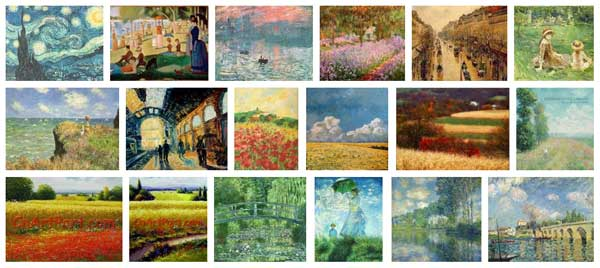Yuri I. Manin in Cyclotomy and analytic geometry over $\mathbb{F}_1$ and Matilde Marcolli in Cyclotomy and endomotives develop a theory of analytic geometry over $\mathbb{F}_1$ based on analytic functions ‘leaking out of roots of unity’. Javier and Oliver depict such functions as ‘thin, but visible brush strokes at roots of 1’ and therefore associate this approach to impressionism. Frow wikipedia : ‘Characteristics of Impressionist paintings include: relatively small, thin, yet visible brush strokes; open composition; emphasis on accurate depiction of light in its changing qualities (often accentuating the effects of the passage of time); common, ordinary subject matter; the inclusion of movement as a crucial element of human perception and experience; and unusual visual angles.’

Connes and Consani : cubismIn On the notion of geometry over $\mathbb{F}_1$ Alain Connes and Katia Consani develop their extension of Soule’s approach. A while ago I’ve done a couple of posts on this here, here and here. Javier and Oliver associate this approach to cubism (a.o. Pablo Picasso and Georges Braque) because of the weird juxtapositions of the simple monoidal pieces in this approach.

Lopez-Pena and Lorscheid : deconstructivismTorified varieties and schemes were introduced by Javier Lopez-Pena and Oliver Lorscheid in Torified varieties and their geometries over $\mathbb{F}_1$ to get lots of examples of varieties over the absolute point in the sense of both Soule and Connes-Consani. Because they were fragmenting schemes into their “fundamental pieces” they associate their approach to deconstructivism.

Another time I’ll sketch my own arty-farty take on all this.Mathblogging.org is a recent initiative and may well become the default starting place to check on the status of the mathematical blogosphere.

Handy, if you want to (re)populate your RSS-aggregator with interesting mathematical blogs, is their graphical presentation of (nearly) all math-blogs ordered by type : group blogs, individual researchers, teachers and educators, journalistic writers, communities, institutions and microblogging (twitter). Links to the last 7 posts are given so you can easily determine whether that particular blog is of interest to you.

The three people behind the project, Felix Breuer, Frederik von Heymann and Peter Krautzberger, welcome you to send them links to (micro)blogs they’ve missed. Surely, there must be a lot more mathematicians with a twitter-account than the few ones listed so far…

Even more convenient is their list of latest posts from their collection, ordered by date. I’ve put that page in my Bookmarks Bar the moment I discovered it! It would be nice, if they could provide an RSS-feed of this list, so that people could place it in their sidebar, replacing old-fashioned and useless blogrolls. The site does provide two feeds, but they are completely useless as they click through to empty pages…

While we’re on the topic of math-blogging, the results of the ‘What should we write about next?’-poll that ran the previous two days on the entry page. Of all people visiting that page, 2.6% left suggestions.

The vast majority (67%) wants more posts on noncommutative geometry. Most of you are craving for introductions (and motivation) accessible to undergraduates (as ‘it’s hard to find quality, updated information on this’). In particular, you want posts giving applications in mathematics (especially number theory), or explaining relationships between different approaches. One person knew exactly how I should go about to achieve the hoped-for accessibility : “As a rule, I’d take what you think would be just right for undergrads, and then trim it down a little more.”

Others want rather specialized posts, such as on ‘connection and parallel transport in noncommutative geometry’ or on ‘trees (per J-L. Loday, M. Aguiar, Connes/Kreimer renormalization (aka Butcher group)), or something completely other tree-related’.

Fortunately, some of you told me it was fine to write about ‘combinatorial games and cool nim stuff, finite simple groups, mathematical history, number theory, arithmetic geometry’, pushed me to go for ‘anything monstrous and moonshiney’ (as if I would know the secrets of the ‘connection between the Mathieu group M24 and the elliptic genus of K3’…) or wrote that ‘various algebraic geometry related posts are always welcome: posts like Mumford’s treasure map‘.

Sunday january 2nd around 18hr NeB-stats went crazy.

Referrals clarified that the post ‘What is the knot associated to a prime?’ was picked up at Reddit/math and remained nr.1 for about a day.

Now, the dust has settled, so let’s learn from the experience.A Reddit-mention is to a blog what doping is to a sporter.

You get an immediate boost in the most competitive of all blog-stats, the number of unique vistors (blue graph), but is doesn’t result in a long-term effect, and, it may even be harmful to more essential blog-stats, such as the average time visitors spend on your site (yellow graph).

For NeB the unique vistors/day fluctuate normally around 300, but peaked to 1295 and 1733 on the ‘Reddit-days’. In contrast, the avg. time on site is normally around 3 minutes, but dropped the same days to 44 and 30 seconds!

Whereas some of the Reddits spend enough time to read the post and comment on it, the vast majority zap from one link to the next. Having monitored the Reddit/math page for two weeks, I’m convinced that post only made it because it was visually pretty good. The average Reddit/math-er is a viewer more than a reader…

So, should I go for shorter, snappier, more visual posts?

Let’s compare Reddits to those coming from the three sites giving NeB most referrals : Google search, MathOverflow and Wikipedia.This is the traffic coming from Reddit/math, as always the blue graph are the unique visitors, the yellow graph their average time on site, blue-scales to the left, yellow-scales to the right.

Here’s the same graph for Google search. The unique visitors/day fluctuate around 50 and their average time on site about 2 minutes.The math-related search terms most used were this month : ‘functor of point approach’, ‘profinite integers’ and ‘bost-connes sytem’.

More rewarding to me are referrals from MathOverflow.The number of visitors depends on whether the MathO-questions made it to the front-page (for example, the 80 visits on december 15, came from the What are dessins d’enfants?-topic getting an extra comment that very day, and having two references to NeB-posts : The best rejected proposal ever and Klein’s dessins d’enfant and the buckyball), but even older MathO-topics give a few referrals a day, and these people sure take their time reading the posts (+ 5 minutes).

Other MathO-topics giving referrals this month were Most intricate and most beautiful structures in mathematics (linking to Looking for F-un), What should be learned in a first serious schemes course? (linking to Mumford’s treasure map (btw. one of the most visited NeB-posts ever)), How much of scheme theory can you visualize? (linking again to Mumford’s treasure map) and Approaches to Riemann hypothesis using methods outside number theory (linking to the Bost-Connes series).

Finally, there’s Wikipediagiving 5 to 10 referrals a day, with a pretty good time-on-site average (around 4 minutes, peaking to 12 minutes). It is rewarding to see NeB-posts referred to in as diverse Wikipedia-topics as ‘Fifteen puzzle’, ‘Field with one element’, ‘Evariste Galois’, ‘ADE classification’, ‘Monster group’, ‘Arithmetic topology’, ‘Dessin d’enfant’, ‘Groupoid’, ‘Belyi’s theorem’, ‘Modular group’, ‘Cubic surface’, ‘Esquisse d’un programme’, ‘N-puzzle’, ‘Shabat polynomial’ and ‘Mathieu group’.

What lesson should be learned from all this data? Should I go for shorter, snappier and more visual posts, or should I focus on the small group of visitors taking their time reading through a longer post, and don’t care about the appallingly high bounce rate the others cause?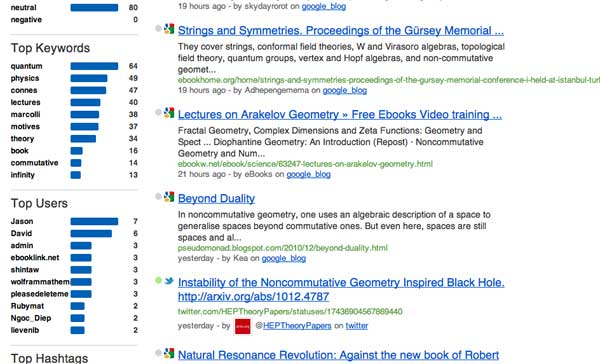SocialMention gives a rather accurate picture of the web-buzz on a specific topic. For this reason I check it irregularly to know what’s going on in noncommutative geometry, at least web-wise.

Yesterday, I noticed two new kids on the block : Jason and David. Their blogs have (so far ) 44 resp. 27 posts, this month alone. My first reaction was: respect!, until I glanced at their content…

David of E-Infinity

Noncommutative geometry has a deplorable track record when it comes to personality-cults and making extra-ordinary claims, but this site beats everything I’ve seen before. Its main mission is to spread the gospel according to E.N.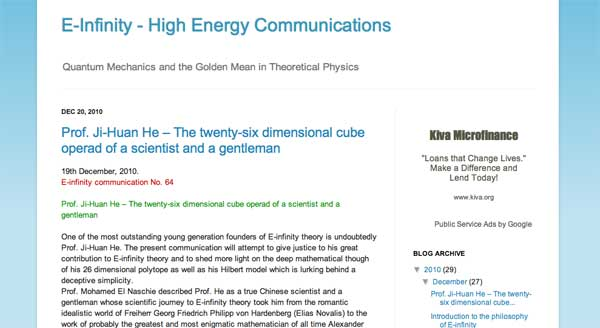A characteristic quote :

“It was no doubt the intention of those well known internet thugs and parasites to distract us from science and derail us from our road. This was the brief given to them by you know who. Never the less we will attempt to give here what can only amount to a summary of the summary of what E. N. considers to be the philosophical background to his theory.”

Jason of the E.N. watch

The blog’s mission statement is to expose the said prophet E.N. as a charlatan.The language used brings us back to the good(?!) old string-war days.

“This is amusing because E. N.’s sockpuppets go on and on about E. N. being a genius polymath with an expert grasp of science, art, history, philosophy and politics. E. N. Watch readers of course know that his knowledge in all areas comes primarily from mass-market popularizations.”

As long as the Connes support-blog and the Rosenberg support-blog remain silent and the Jasons and Davids of this world run the online ncg-show, it is probably better to drop the topic here.